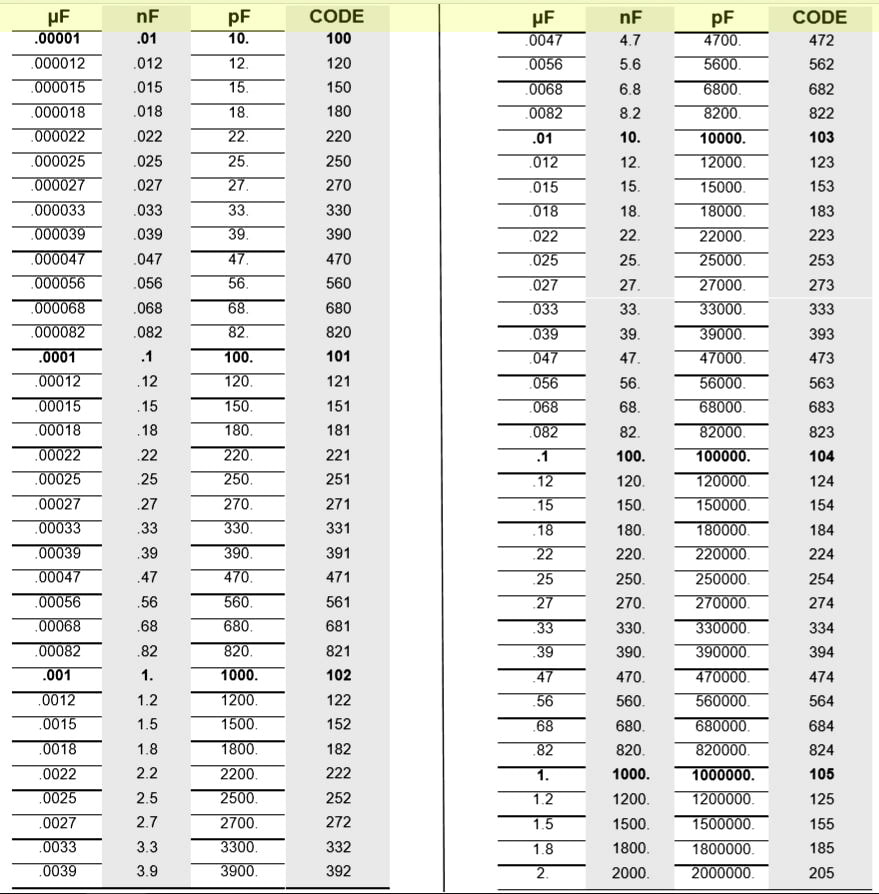## Capacitor Code Calculation with chart

In this article, I have explained how to calculate the capacitance value from the 3-digit capacitor code. For the ceramic capacitors, a 3-digit code marked on the capacitor indicates their capacitance value.

### What is Ceramic Capacitor

Ceramic capacitors are the fixed-value capacitors where the dielectric is made of ceramic materials. For any ceramic capacitors, there are two or more alternating layers of ceramic and the metal layer acting as the electrodes.

### Capacitor Codes Chart:chart for capacitor codes with capacitance value in pF and nF

### How to calculate capacitor code 104

The most common code uses the first digit, second digit, and multiplier scheme.

In this picture, I have shown how to get the capacitance value from the 104 capacitor code.

To get the capacitance value first write down the first and second digit. The third digit indicates the number of zeros you have to write after the first two-digit.

For code 104, the third digit is 4, so you have to write 0000 (4 zeros) after the 10 (first two-digit).

So the capacitance value for 104 will be 100000 picofarads or 100 nanofarads or 0.1 microfarad.

### Tolerance Marking Code

#### More Examples:

For some capacitors the capacitance value listed very plainly.

As you can see in the picture for the 22pF the 22K is marked on the capacitor. (K indicate tolerance is 10%)

### Measuring Capacitance with Multimeter

You can also use a multimeter to test the capacitance value of capacitors. Here I am testing the 155J ceramic capacitor. In the multimeter, you can the capacitance value is 1.5uF.

Please share your feedback on this tutorial video on capacitors and also let me know if you have any queries.

You can also visit our YouTube channel for more such useful tutorials on basic electronics.

I hope you have liked this tutorial, Thank you for your time.